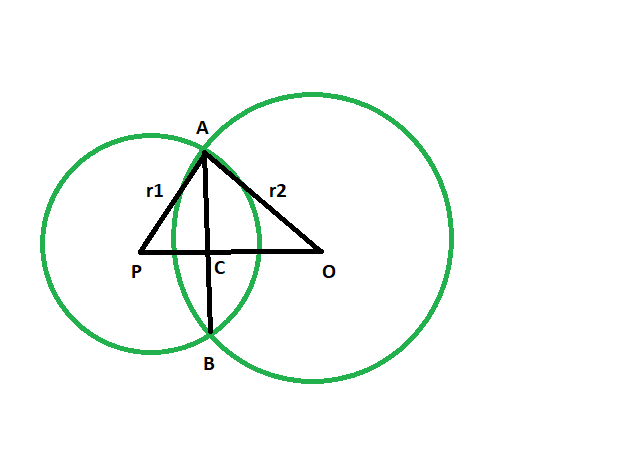# Distance between centers of two intersecting circles if the radii and common chord length is given

• Last Updated : 05 Nov, 2021

Given are two circles, with given radii, which intersect each other and have a common chord. The length of the common chord is given. The task is to find the distance between the center of the two circles.

Examples:

Attention reader! Don’t stop learning now. Get hold of all the important DSA concepts with the DSA Self Paced Course at a student-friendly price and become industry ready.  To complete your preparation from learning a language to DS Algo and many more,  please refer Complete Interview Preparation Course.

In case you wish to attend live classes with experts, please refer DSA Live Classes for Working Professionals and Competitive Programming Live for Students.

```Input:  r1 = 24, r2 = 37, x = 40
Output: 44

Input: r1 = 14, r2 = 7, x = 10
Output: 17
```Approach:

• let the length of common chord AB = x
• Let the radius of the circle with center O is OA = r2
• Radius of circle with center P is AP = r1
• From the figure, OP is perpendicular AB
AC = CB
AC = x/2 (Since AB = x)
• In triangle ACP,
AP^2 = PC^2+ AC^2 [By Pythagoras theorem]
r1^2 = PC^2 + (x/2)^2
PC^2 = r1^2 – x^2/4
• Consider triangle ACO
r2^2 = OC^2+ AC^2[By Pythagoras theorem]
r2^2 = OC^2+ (x/2)^2
OC^2 = r2^2 – x^2/4
• From the figure, OP = OC + PC
OP = √( r1^2 – x^2/4 ) + √(r2^2 – x^2/4)

Distance between the centers = sqrt((radius of one circle)^2 – (half of the length of the common chord )^2) + sqrt((radius of the second circle)^2 – (half of the length of the common chord )^2)

Below is the implementation of the above approach:

## C++

 `// C++ program to find``// the distance between centers``// of two intersecting circles``// if the radii and common chord length is given`` ` `#include ``using` `namespace` `std;`` ` `void` `distcenter(``int` `r1, ``int` `r2, ``int` `x)``{``    ``int` `z = ``sqrt``((r1 * r1)``                 ``- (x / 2 * x / 2))``            ``+ ``sqrt``((r2 * r2)``                   ``- (x / 2 * x / 2));`` ` `    ``cout << ``"distance between the"``         ``<< ``" centers is "``         ``<< z << endl;``}`` ` `// Driver code``int` `main()``{``    ``int` `r1 = 24, r2 = 37, x = 40;``    ``distcenter(r1, r2, x);``    ``return` `0;``}`

## Java

 `// Java program to find``// the distance between centers``// of two intersecting circles``// if the radii and common chord length is given``import` `java.lang.Math; ``import` `java.io.*;`` ` `class` `GFG {``     ` `static` `double` `distcenter(``int` `r1, ``int` `r2, ``int` `x)``{``    ``double` `z = (Math.sqrt((r1 * r1)``                ``- (x / ``2` `* x / ``2``)))``            ``+ (Math.sqrt((r2 * r2)``                ``- (x / ``2` `* x / ``2``)));`` ` `    ``System.out.println (``"distance between the"` `+``                        ``" centers is "``+ (``int``)z );``    ``return` `0``;``}`` ` `// Driver code``public` `static` `void` `main (String[] args) ``{``    ``int` `r1 = ``24``, r2 = ``37``, x = ``40``;``    ``distcenter(r1, r2, x);``}``}`` ` `// This code is contributed by jit_t.`

## Python3

 `     ` `# Python program to find``# the distance between centers``# of two intersecting circles``# if the radii and common chord length is given`` ` `def` `distcenter(r1, r2, x):``    ``z ``=` `(((r1 ``*` `r1) ``-` `(x ``/` `2` `*` `x ``/` `2``))``*``*``(``1``/``2``)) ``+``\``    ``(((r2 ``*` `r2)``-` `(x ``/` `2` `*` `x ``/` `2``))``*``*``(``1``/``2``));`` ` `    ``print``(``"distance between thecenters is "``,end``=``"");``    ``print``(``int``(z));`` ` `# Driver code``r1 ``=` `24``; r2 ``=` `37``; x ``=` `40``;``distcenter(r1, r2, x);`` ` `# This code has been contributed by 29AjayKumar`

## C#

 `// C# program to find``// the distance between centers``// of two intersecting circles``// if the radii and common chord length is given``using` `System;`` ` `class` `GFG``{``         ` `static` `double` `distcenter(``int` `r1, ``int` `r2, ``int` `x)``{``    ``double` `z = (Math.Sqrt((r1 * r1)``                ``- (x / 2 * x / 2)))``            ``+ (Math.Sqrt((r2 * r2)``                ``- (x / 2 * x / 2)));`` ` `    ``Console.WriteLine(``"distance between the"` `+``                        ``" centers is "``+ (``int``)z );``    ``return` `0;``}`` ` `// Driver code``static` `public` `void` `Main ()``{``    ``int` `r1 = 24, r2 = 37, x = 40;``    ``distcenter(r1, r2, x);``}``}`` ` `// This code is contributed by jit_t`
Output:
```distance between the centers is 44
```

My Personal Notes arrow_drop_up